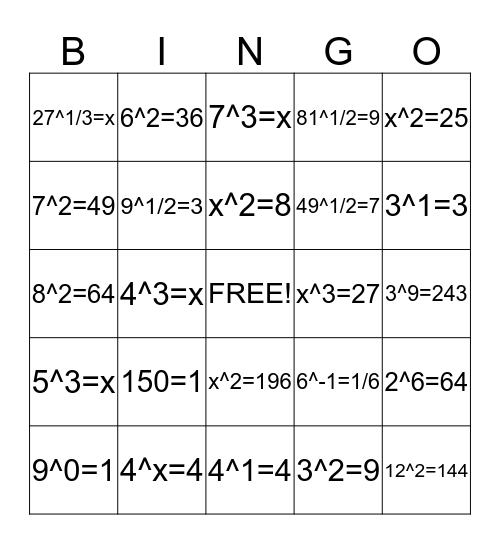# ExponentialsThis bingo card has a free space and 24 words: 150=1, 4^x=4, x^2=8, 9^0=1, x^2=25, 27^1/3=x, 7^2=49, 6^2=36, x^3=27, x^2=196, 49^1/2=7, 8^2=64, 6^-1=1/6, 2^6=64, 9^1/2=3, 3^2=9, 3^9=243, 7^3=x, 3^1=3, 4^3=x, 12^2=144, 5^3=x, 81^1/2=9 and 4^1=4.

## Play Online